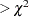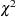The FACTOR Procedure
 Displayed Output

PROC FACTOR output includes the following.

• Input data type, numbers of records read and used for raw data input, number of observations (NOBS=) set in the PROC FACTOR statements, and the number of observations used in significance tests

• Mean and Std Dev (standard deviation) of each variable and the number of observations, if you specify the SIMPLE option

• Correlations, if you specify the CORR option

• Inverse Correlation Matrix, if you specify the ALL option

• Partial Correlations Controlling all other Variables (negative anti-image correlations), if you specify the MSA option. If the data are appropriate for the common factor model, the partial correlations should be small.

• Kaiser’s Measure of Sampling Adequacy (Kaiser; 1970; Kaiser and Rice; 1974; Cerny and Kaiser; 1977), both overall and for each variable, if you specify the MSA option. The MSA is a summary of how small the partial correlations are relative to the ordinary correlations. Values greater than 0.8 can be considered good. Values less than 0.5 require remedial action, either by deleting the offending variables or by including other variables related to the offenders.

• Prior Communality Estimates, unless 1.0s are used or unless you specify the METHOD=IMAGE, METHOD=HARRIS, METHOD=PATTERN, or METHOD=SCORE option

• Squared Multiple Correlations of each variable with all the other variables, if you specify the METHOD=IMAGE or METHOD=HARRIS option

• Image Coefficients, if you specify the METHOD=IMAGE option

• Image Covariance Matrix, if you specify the METHOD=IMAGE option

• Preliminary Eigenvalues based on the prior communalities, if you specify the METHOD=PRINIT, METHOD=ALPHA, METHOD=ML, or METHOD=ULS option. The table produced includes the Total and the Average of the eigenvalues, the Difference between successive eigenvalues, the Proportion of variation represented, and the Cumulative proportion of variation.

• the number of factors that are retained, unless you specify the METHOD=PATTERN or METHOD=SCORE option

• the Scree Plot of Eigenvalues, if you specify the SCREE option. The preliminary eigenvalues are used if you specify the METHOD=PRINIT, METHOD=ALPHA, METHOD=ML, or METHOD=ULS option.

• the iteration history, if you specify the METHOD=PRINIT, METHOD=ALPHA, METHOD=ML, or METHOD=ULS option. The table produced contains the iteration number (Iter); the Criterion being optimized (Jöreskog; 1977); the Ridge value for the iteration if you specify the METHOD=ML or METHOD=ULS option; the maximum Change in any communality estimate; and the Communalities.

• Significance tests, if you specify the option METHOD=ML, including Bartlett’s chi-square, df, and Probfor H: No common factors and H: factors retained are sufficient to explain the correlations. The Htest for no common factors is equivalent to Bartlett’s test of sphericity. The variables should have an approximate multivariate normal distribution for the probability levels to be valid. Lawley and Maxwell (1971) suggest that the number of observations should exceed the number of variables by 50 or more, although Geweke and Singleton (1980) claim that as few as 10 observations are adequate with five variables and one common factor. Certain regularity conditions must also be satisfied for Bartlett’stest to be valid (Geweke and Singleton; 1980), but in practice these conditions usually are satisfied. The notation Prob>chi**2 means "the probability under the null hypothesis of obtaining a greaterstatistic than that observed." The chi-square value is displayed with and without Bartlett’s correction.

• Akaike’s Information Criterion, if you specify the METHOD=ML option. Akaike’s information criterion (AIC) (Akaike; 1973, 1974, 1987) is a general criterion for estimating the best number of parameters to include in a model when maximum likelihood estimation is used. The number of factors that yields the smallest value of AIC is considered best. Like the chi-square test, AIC tends to include factors that are statistically significant but inconsequential for practical purposes.

• Schwarz’s Bayesian Criterion, if you specify the METHOD=ML option. Schwarz’s Bayesian Criterion (SBC) (Schwarz; 1978) is another criterion, similar to AIC, for determining the best number of parameters. The number of factors that yields the smallest value of SBC is considered best; SBC seems to be less inclined to include trivial factors than either AIC or the chi-square test.

• Tucker and Lewis’s Reliability Coefficient, if you specify the METHOD=ML option (Tucker and Lewis; 1973)

• Squared Canonical Correlations, if you specify the METHOD=ML option. These are the same as the squared multiple correlations for predicting each factor from the variables.

• Coefficient Alpha for Each Factor, if you specify the METHOD=ALPHA option

• Eigenvectors, if you specify the EIGENVECTORS or ALL option, unless you also specify the METHOD=PATTERN or METHOD=SCORE option

• Eigenvalues of the (Weighted) (Reduced) (Image) Correlation or Covariance Matrix, unless you specify the METHOD=PATTERN or METHOD=SCORE option. Included are the Total and the Average of the eigenvalues, the Difference between successive eigenvalues, the Proportion of variation represented, and the Cumulative proportion of variation.

• the Factor Pattern, which is equal to both the matrix of standardized regression coefficients for predicting variables from common factors and the matrix of correlations between variables and common factors since the extracted factors are uncorrelated. Standard error estimates are included if the SE option is specified with METHOD=ML. Confidence limits and coverage displays are included if COVER= option is specified with METHOD=ML.

• Variance explained by each factor, both Weighted and Unweighted, if variable weights are used

• Final Communality Estimates, including the Total communality; or Final Communality Estimates and Variable Weights, including the Total communality, both Weighted and Unweighted, if variable weights are used. Final communality estimates are the squared multiple correlations for predicting the variables from the estimated factors, and they can be obtained by taking the sum of squares of each row of the factor pattern, or a weighted sum of squares if variable weights are used.

• Residual Correlations with Uniqueness on the Diagonal, if you specify the RESIDUAL or ALL option

• Root Mean Square Off-diagonal Residuals, both Over-all and for each variable, if you specify the RESIDUAL or ALL option

• Partial Correlations Controlling Factors, if you specify the RESIDUAL or ALL option

• Root Mean Square Off-diagonal Partials, both Over-all and for each variable, if you specify the RESIDUAL or ALL option

• Plots of Factor Pattern for unrotated factors, if you specify the PREPLOT option. The number of plots is determined by the NPLOT= option.

• Variable Weights for Rotation, if you specify the NORM=WEIGHT option

• Factor Weights for Rotation, if you specify the HKPOWER= option

• Orthogonal Transformation Matrix, if you request an orthogonal rotation

• Rotated Factor Pattern, if you request an orthogonal rotation. Standard error estimates are included if the SE option is specified with METHOD=ML. Confidence limits and coverage displays are included if COVER= option is specified with METHOD=ML.

• Variance explained by each factor after rotation. If you request an orthogonal rotation and if variable weights are used, both weighted and unweighted values are produced.

• Target Matrix for Procrustean Transformation, if you specify the ROTATE=PROMAX or ROTATE=PROCRUSTES option

• the Procrustean Transformation Matrix, if you specify the ROTATE=PROMAX or ROTATE=PROCRUSTES option

• the Normalized Oblique Transformation Matrix, if you request an oblique rotation, which, for the option ROTATE=PROMAX, is the product of the prerotation and the Procrustes rotation

• Inter-factor Correlations, if you specify an oblique rotation. Standard error estimates are included if the SE option is specified with METHOD=ML. Confidence limits and coverage displays are included if COVER= option is specified with METHOD=ML.

• Rotated Factor Pattern (Std Reg Coefs), if you specify an oblique rotation, giving standardized regression coefficients for predicting the variables from the factors. Standard error estimates are included if the SE option is specified with METHOD=ML. Confidence limits and coverage displays are included if COVER= option is specified with METHOD=ML.

• Reference Axis Correlations if you specify an oblique rotation. These are the partial correlations between the primary factors when all factors other than the two being correlated are partialed out.

• Reference Structure (Semipartial Correlations), if you request an oblique rotation. The reference structure is the matrix of semipartial correlations (Kerlinger and Pedhazur; 1973) between variables and common factors, removing from each common factor the effects of other common factors. If the common factors are uncorrelated, the reference structure is equal to the factor pattern.

• Variance explained by each factor eliminating the effects of all other factors, if you specify an oblique rotation. Both Weighted and Unweighted values are produced if variable weights are used. These variances are equal to the (weighted) sum of the squared elements of the reference structure corresponding to each factor.

• Factor Structure (Correlations), if you request an oblique rotation. The (primary) factor structure is the matrix of correlations between variables and common factors. If the common factors are uncorrelated, the factor structure is equal to the factor pattern. Standard error estimates are included if the SE option is specified with METHOD=ML. Confidence limits and coverage displays are included if COVER= option is specified with METHOD=ML.

• Variance explained by each factor ignoring the effects of all other factors, if you request an oblique rotation. Both Weighted and Unweighted values are produced if variable weights are used. These variances are equal to the (weighted) sum of the squared elements of the factor structure corresponding to each factor.

• Final Communality Estimates for the rotated factors if you specify the ROTATE= option. The estimates should equal the unrotated communalities.

• Squared Multiple Correlations of the Variables with Each Factor, if you specify the SCORE or ALL option, except for unrotated principal components

• Standardized Scoring Coefficients, if you specify the SCORE or ALL option

• Plots of the Factor Pattern for rotated factors, if you specify the PLOT option and you request an orthogonal rotation. The number of plots is determined by the NPLOT= option.

• Plots of the Reference Structure for rotated factors, if you specify the PLOT option and you request an oblique rotation. The number of plots is determined by the NPLOT= option. Included are the Reference Axis Correlation and the Angle between the Reference Axes for each pair of factors plotted.

If you specify the ROTATE=PROMAX option, the output includes results for both the prerotation and the Procrustes rotation.Previous Page | Next Page | Top of Page